• plot T=table2array(t) 导入的表格为table格式...title('电机转矩特性') 标题 axis([xmin xmax ymin ymax]) 坐标范围 contour(s,T,XL)等高线图 [c,h]=contour(s,T,XL); set(h,'ShowText','on') 显示等高线的值 ...
plot
T=table2array(t)
导入的表格为table格式，转化为array数组
xlabel('电机转速');
起名字
title('电机转矩特性')
标题
axis([xmin xmax ymin ymax])
坐标范围
contour(s,T,XL)等高线图
 [c,h]=contour(s,T,XL);
set(h,'ShowText','on')

显示等高线的值


展开全文• 基本绘图指令plotPolt命令MATLAB中最常使用也是最基础的画图函数。语法：Plot(x,y)是绘制以x为横坐标，y为纵坐标的二维曲线Plot(y)是绘制以y为纵坐标的二维坐标曲线，这是默认横坐标为1、2、3…...
 这里介绍了基本的二维图像的绘制，包括以下内容：基本绘图指令plot的用法；多条曲线绘图；不同曲线进行标注；曲线样式设置；横纵坐标以及标题设置；在图像上添加文本、箭头和直线。 1. 基本绘图指令plotPolt命令是MATLAB中最常使用也是最基础的画图函数。语法：Plot(x,y)是绘制以x为横坐标，y为纵坐标的二维曲线Plot(y)是绘制以y为纵坐标的二维坐标曲线，这是默认横坐标为1、2、3…例： plot (cos(0:pi/20:2*pi))分析：这里用的是上面的语法的第二种，绘制关于函数cos(0:pi/20:2*pi)的图像。运行结果为：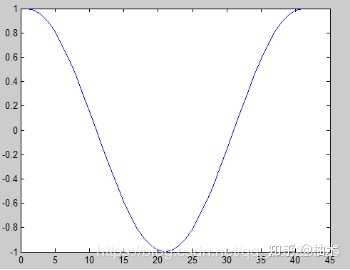2. hold on 和hold off指令如果直接执行以下指令：plot (cos(0:pi/20:2*pi));plot (sin(0:pi/20:2*pi));那么最终只会显示后一个画图的结果，是因为MATLAB中会将前面的作图清除。如果我们需要显示两个图，就需要使用hold on命令，具体操作如下：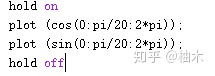运行结果为：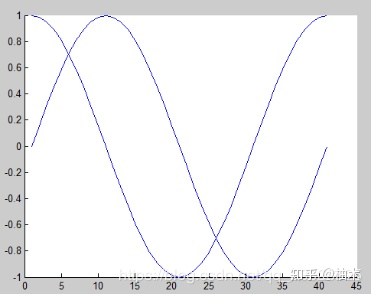3. plot styleplot命令可以设置曲线的线段类型，颜色以及数据点形等，具体参见下表：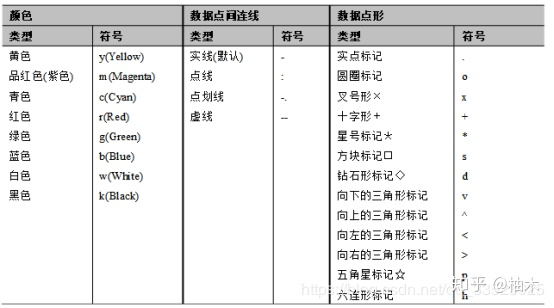语法：Plot(x,y,’str’);尝试进行一些相关操作：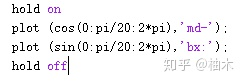运行结果如下：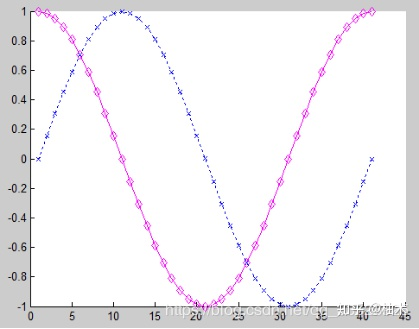4. legend()指令当画了多个图像在一个图中，我们需要对曲线进行标注，才能将图像区分开来，这时就用到了legend这个语句。语法：Legend(‘str1’,’str2’, ...,参数)其中参数字符串含义如下：0 尽量不与数据冲突，放于最优位置1 放在右上角2 放在左上角3 放在左下角4 放在右下角-1 放在图形视窗外的右上方举例：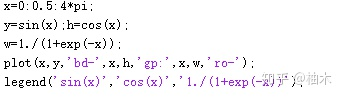运行结果如下：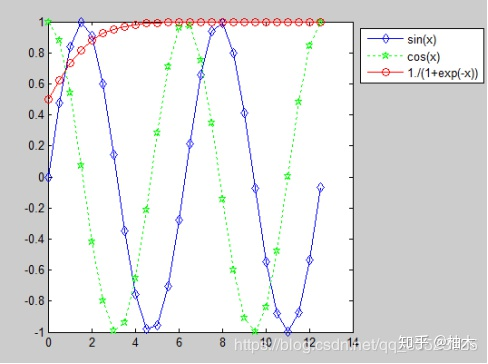5. title()和label()指令在绘图时需要对图像进行标题命名以及横纵坐标的显示，这里就用到了title()和label()这两个指令。举例说明：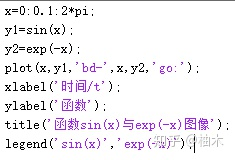运行结果如下：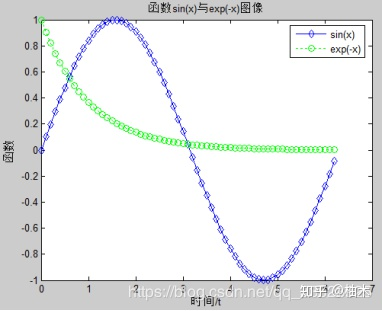6. text()与annotation()指令我们在作图的时候可以在图片上进行一些文字描述，这里会使用到LaTeX，具体的用法通过下面的例子进行说明。例：画出函数的图像并将函数表达式标明在图像中。分析：①关于text的语法：text(0.25,2.5,str,'Interpreter','latex');前面是显示text的位置设置，在(0.25,2.5)这个位置，str是显示的内容，后面的部分固定。②积分书写这里子串的书写比较复杂，关于积分号的书写为int{0}^{2};积分运算写为 in_t{0}^{2} x^2sin(x) dx ，整个字符串格式参见程序。③关于annotation我们可以在图片在添加一个箭头，具体实现如下：annotation('arrow','x',[0.32,0.5],'Y',[0.6,0.4])设置x和y可以设置箭头的位置，这里表示从（0.32,0.6）到（0.5,0.4）位置的有向箭头。④关于line()函数：由于是定积分，是对应图像的一块面积，从0到2，要在图像中画一条线，这里需要使用line()函数，具体实现如下：line([2,2],[0,2.^2.*sin(2)]);表示从（2，0）到（2，2.^2.*sin(2)）的一条直线。具体实现如下：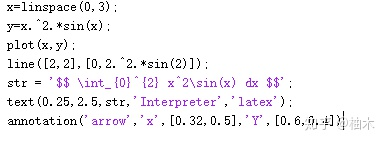运行结果如下：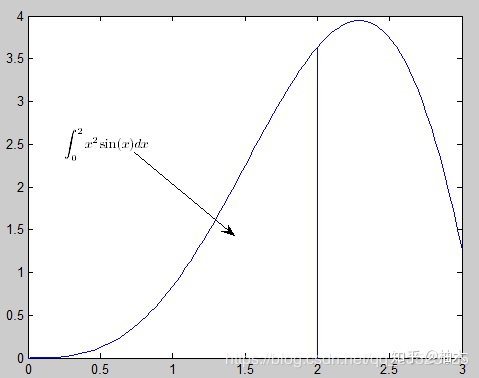展开全文• 1.在命令窗口输入命令时，可以不必每输入一条命令就按enter键执行，可以在输入几行后一同运行。方法是：换行时，只要在按住<shift>键的同时按<enter>键即可，否则matlab就会执行上面输入的所有语句。2....
1.在命令窗口输入命令时，可以不必每输入一条命令就按enter键执行，可以在输入几行后一同运行。方法是：换行时，只要在按住<shift>键的同时按<enter>键即可，否则matlab就会执行上面输入的所有语句。2.如何将数据文件导入matlab中并画图：点击file中import data,然后就可以导入文件，然后再workspace里面，点击该数据文件，就可以出现以下图形：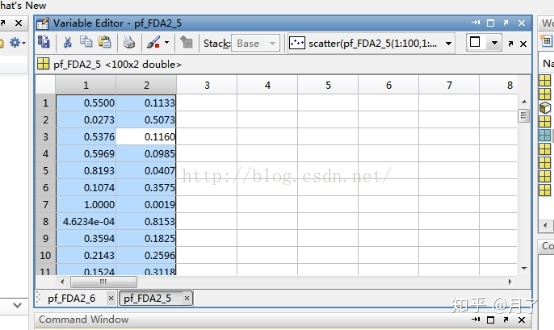选择该两列，就可以看到在上面有一个scatter然后点击，就可以出现以下图形：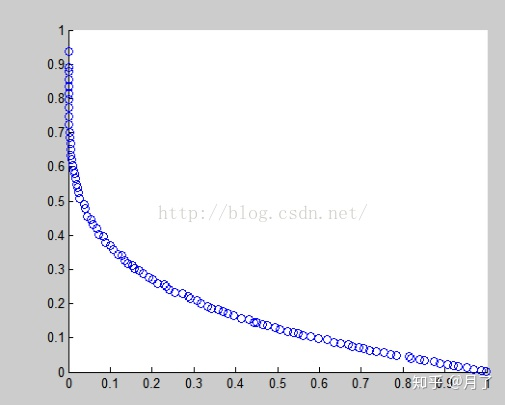3.分号“;”的作用：用做矩阵（数组）的行间分隔符，用做不显示计算结果的命令。4.稀疏矩阵 定义：矩阵大部分元素是0，少部分元素是非零元素，这种矩阵是稀疏矩阵。S=sparse(A);full(S);nnz(S);spy(S);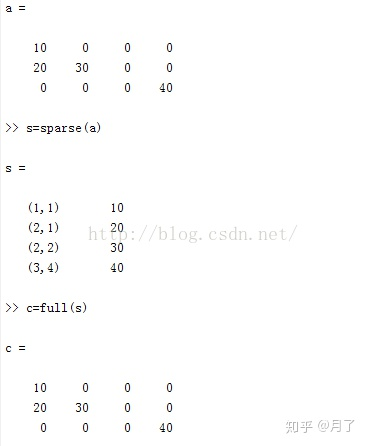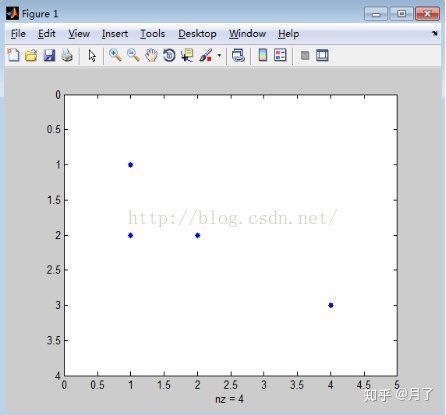spy(s,,‘*’，16） 表示用’*’号显示，大小为16。如图：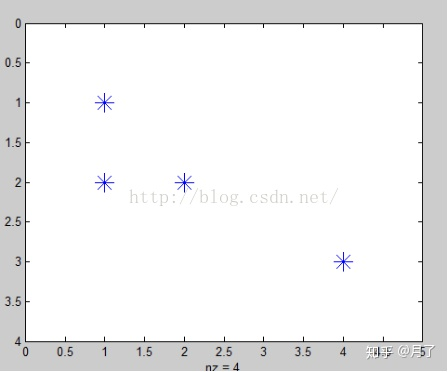5.注意矩阵的乘法A*B和A.*B的区别：A*B就是A与B的直接相乘，如果是A.*B就是A与B中的对应项相乘。 “./”也是一样的，就是对应项相除。6.奇异矩阵就是方阵并且行列式为0的矩阵。7.二维图像绘制（1）基本函数绘制  plot 既可以绘制实线，也可以绘制点线。 <1> plot(X,Y)  %X,Y为同维向量时，绘制以X、Y元素为横，纵坐标的一条线；X为列向量，Y为矩阵时，按Y列绘制多条不同颜色的曲线，X为这些曲线共同的横坐标。<2> plot(X,Y,LineSpec) %参数LineSpec用于指出线条的类型，标记符号和颜色。<3> plot(X1,Y1,LineSpec1,X2,Y2,LineSpec2...) %当Xi和Yi成对出现时，将分别按顺序取两数据Xi和Yi进行画图。<4> plot(..., ‘PropertyName’,PropertyValue,...)%对图形对象中指定的属性进行设置。For example:  x=0:0.1:2;  y=1+exp(x);  Plot(x,y, ‘-+b’)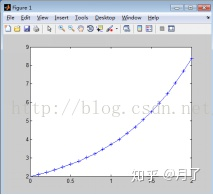其中，‘-’表示实线，‘+’表示点类型，b表示蓝色。t=[-pi:pi/100:2*pi]’;k=1:6; y=sin(t)*k;Plot(t,y)   %y是矩阵形式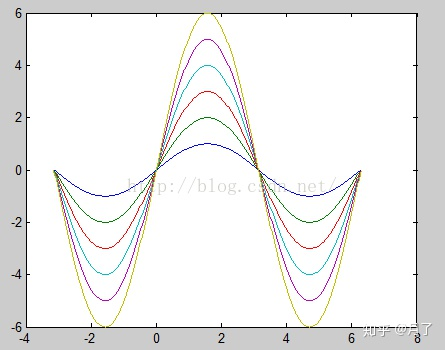（2）图形标注 <1> 坐标轴名 格式：xlabel(‘string’)        Ylabel(‘string’) 分别给x轴和y轴添加标签 <2> 图名格式：title(‘string’)  在当前坐标轴上方正中央放置字符串string做为标题。 <3> 图例格式：legend(‘string1’,’string2’,...) 用指定文字给当前坐标轴添加图例。For example:t=0:pi/100:pi;y1=sin(t);y2=sin(-t);y3=sin(t).*sin(5*t);plot(t,y1, ‘-.r’,t,y2, ‘-.k’,t,y3, ‘-bo’)xlabel(‘时间’);ylabel(‘幅度’);Title(‘波形及包络线’);Legend(‘y=sint’, ‘y=-sint’, ‘y=sinsin5t’)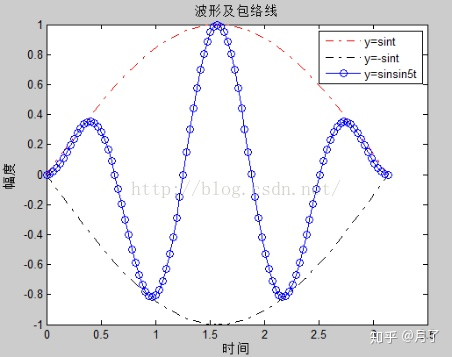上面的图形标注都是使用标注函数直接写在程序中的，当执行程序后，图形中会自动添加图形标注。但是我们也可以根据图形菜单进行标注，打开图形窗口（Figure)菜单栏中的insert菜单，这时候就可以在图像上添加标注。（3）图像控制命令  <1>clf   %清除所有当前图像窗口（4）迭加绘图  <1>hold on   %保留当前图像与当前坐标轴的属性值，使用后面的图形命令只能在当前存在的坐标轴中增加图形。  <2>hold off   %在绘制新图形之前，重新设置坐标轴的属性为默认值，关闭hold on功能。  <3>hold     %在on和off之间切换，即在增加图形和覆盖图形之间切换。  <4>hold all   %保留当前颜色和线型，在绘制随后的图形时使用当前颜色和线型。 For example: x=linspace(0,2*pi,60);y=sin(x); plot(x,y,’b’); xlabel(‘自变量’),ylabel(‘因变量’); Hold on; z=0.5*sin(x); plot(x,z,’k:’); legend(‘y=sin(x)’,’z=0.5*sin(x)’); hold off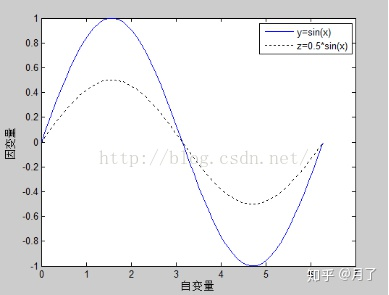（5）多子图 在同一图形框内布置几幅独立的子图。 格式：subplot(m,n,k)   %将一个图形窗口分成m*n个小窗口（子图），k是子图的编号。序号原则是：左上方的为第一幅，然后向右、向下依次排好。           subplot(‘Position’,[left bottom width height])  %在由4个元素指定的位置上创建坐标轴。   x=-4:0.1:4;   subplot(2,2,1);   y1=1/sqrt(2*pi)*exp(-1/2*x.^2),plot(x,y1);   xlabel('变量x'),ylabel('变量密度y'),title('正太分布N(0,1)');   subplot(2,2,2);   y2=1/sqrt(2*pi)/2*exp(-1/2/4*x.^2),plot(x,y2);   xlabel('变量x'),ylabel('概率密度y')   title('正太分布N(0,4)');   subplot(2,2,3);   y3=1/sqrt(2*pi)/0.5*exp(-1/2/(0.5^2)*(x-1).^2),plot(x,y3);   xlabel('变量x'),ylabel('概率密度y'),title('正太分布N(1,1/4)');   subplot(2,2,4);   y4=1/sqrt(2*pi)/0.5*exp(-1/2/(0.5^2)*(x+1).^2),plot(x,y4)    xlabel('变量x'),ylabel('概率密度y'),title('正太分布N(-1,1/4)')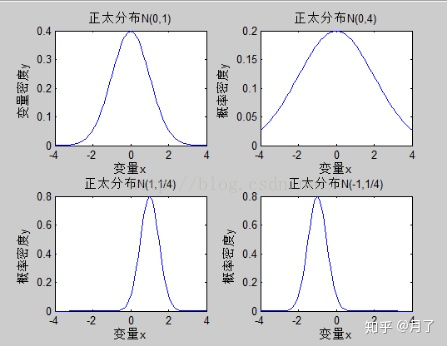（6）多个图形窗口 格式： Figure(n)    %创建新的图形窗口或显示当前图形窗口。n是这个窗口的编号，figure(1)是默认值，不需要声明。>> t=-4:0.1:4;x=sin(t);plot(t,x,'b^')>> t=-4:0.1:4;x=sin(t);plot(t,x,'b^')>> xlabel('t');ylabel('x');title('函数x=sint的图形');>> figure(2)>> y=cos(t);plot(t,y,'kp');>> xlabel('t');ylabel('y');title('函数y=cost的图形');>> figure(3)>> z=sin(t).*cos(t);plot(t,z,'kh')>> xlabel('t');ylabel('z');title('函数z=sincost的图形')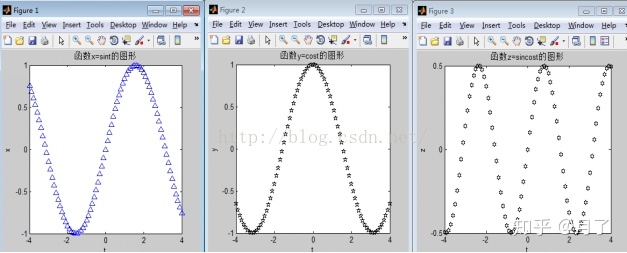（7）对数比例坐标轴     loglog(x,y)  %双对数坐标，在x轴，y轴按对数比例绘制二维图形。     Semilogx   %半对数坐标，在x轴按对数比例，y轴按线型比例绘制二维图形。     Semilogy   %半对数坐标，在y轴按对数比例，x轴按线型比例绘制二维图形。 x=linspace(0,5,20);y=exp(x);>> subplot(2,2,1),plot(x,y),xlabel('x'),ylabel('y');>> subplot(2,2,2),loglog(x,y),xlabel('logx'),ylabel('logy');>> subplot(2,2,3),semilogx(x,y),xlabel('logx'),ylabel('y');>> subplot(2,2,4),semilogy(x,y),xlabel('x'),ylabel('logy');>>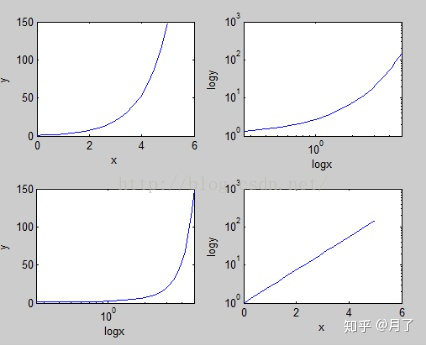（8）双纵坐标图 格式：plotyy(x1,y1,x2,y2)     %绘制双纵坐标二维图形，x1和y1所对应的图形的纵坐标标注在图形的左边，x2和y2所对应图形的纵坐标标注在图形的右边。>> x=0:0.1:4;y=x.*sin(x);s=sin(x)-x.*cos(x);>> plotyy(x,y,x,s); >> text(0.5,0,'fontsize{14}ity=xsinx')>>text(2.5,3.5,['fontsize{14}its=','{fontsize{16}  int_{fontsize{8}0}^{ x}}','fontsize{14}itxsinxdx'])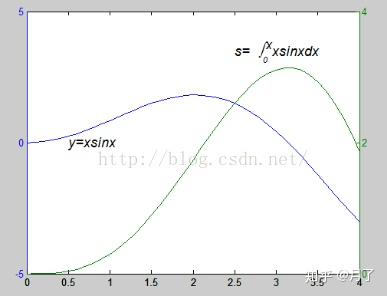(9) 泛函绘图 泛函函数fplot(‘function’,limits,LineSpec)           fplot(‘function’,limits,LineSpec,tol) 说明： （1）在指定的范围limits内绘制出函数名为function的一元函数图像，其中limits是一个指定x轴的向量[xmin xmax],或者是x轴和y轴的范围向量[xmin xmax ymin ymax]。Tol为相对允许误差，默认值为2e-3.   (2)函数function必须是M函数文件或者只包含一个变量x的函数字符串。   (3)用指定的线型LineSpec绘制出函数function.For example: fplot('exp(2*x)',[0 2],'o')图像为：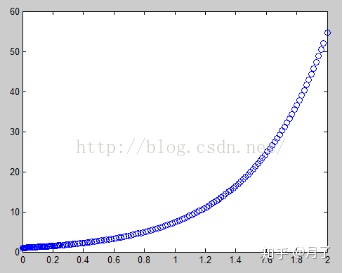fplot(‘[sin(x),cos(x)]’,[0 2*pi-1.2 1.2],’-*’,1e-3)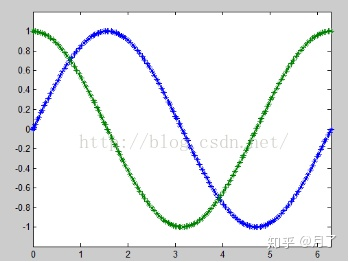(10) 简易函数绘图
展开全文• 一、本文主要内容和相关参考博客 参考了xticklabel和xtick在matlab里的区别 和label ‘ytick’的设置这两个网址。 matlab在画图时会自动调整坐标轴间隔的大小，但是我们可以使用如set(gca,'... "plot","title","x...
一、本文主要内容和相关参考博客

参考了xticklabel和xtick在matlab里的区别  和 label ‘ytick’的设置这两个网址。
matlab在画图时会自动调整坐标轴间隔的大小，但是我们可以使用如set(gca,'ytick',ylabel_pos,'yticklabel',ylabel_str)的函数来实现自己调整间隔以画出我们满意的图。
"plot","title","xlabel","ylabel","grid on","figure","hold on","linspace"等基本命令的使用。
二、相关实例

反正弦函数和反余弦函数图像的绘制。
1)要明确函数表达式和定义域，因为他们传入到plot等画图函数中。

y1 = arcsin(x)  x[-1,1];    y2 = arccos(x)  x[-1,1];

2)画图代码如下：

%%
x = linspace(-1,1,100);%%返回包含 x1 和 x2 之间的 100 个等间距点的行向量。
y = asin(x);
plot(x,y);
title('反正弦函数图像');
grid on;
xlabel('x');
ylabel('y');
figure;
x = linspace(-1,1,100);
y = acos(x);
plot(x,y);
title('反余弦函数图像');
grid on;
xlabel('x');
ylabel('y');

3）title: 设置标题；grid on : 绘制网格；xlabel 和ylabel: 设置横纵坐标；figure:另外再开启一张图。正是因为有了figure命令的存在，最终得到的结果才有两幅图，如下：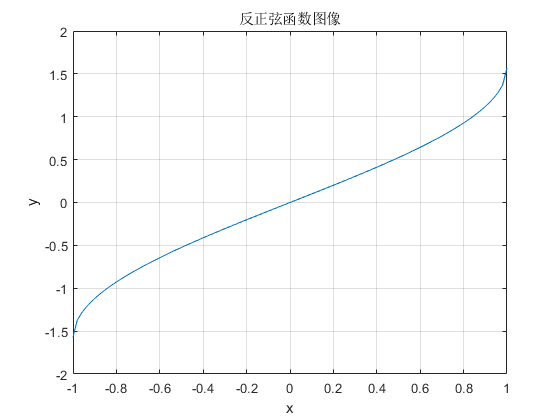图1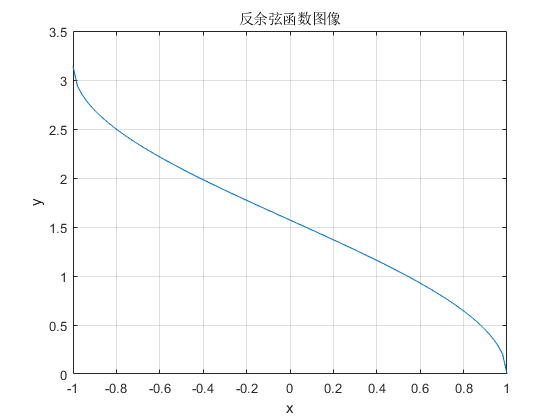图2

4）当然也可以将两个函数画在同一张图片，使用 hold on命令即可，同时使用这个set(gca,'ytick',ylabel_pos,'yticklabel',ylabel_str);语句，其中‘ylabel_pos’是用来来设置y轴的间隔大小，'ylabel_str'是用来描述各个间隔的字符，ylabel_pos和ylabel_str这两个数组长度尽量保持一致。具体可见上文引用的第一个链接，代码如下。

x = linspace(-1,1);
y1 = asin(x);

plot(x,y1);
hold on;
y2 = acos(x);
plot(x,y2);
ylabel_pos = -pi:pi/4:2*pi;
ylabel_str = {'-pi','-3/4*pi','-2/4*pi','-1/4*pi','0','1/4*pi','2/4*pi','3/4*pi','pi','5/4*pi'};
%set(gca,'ytick',-pi:pi/4:2*pi);%set语句必须放在画图函数的后面
set(gca,'ytick',ylabel_pos,'yticklabel',ylabel_str);
title('asin(x)与acos(x)图像比较');
grid on;
xlabel('x');
ylabel('y');
legend('asin(x)','acos(x)');

未使用set(gca,'ytick',ylabel_pos,'yticklabel',ylabel_str);得到以下图像。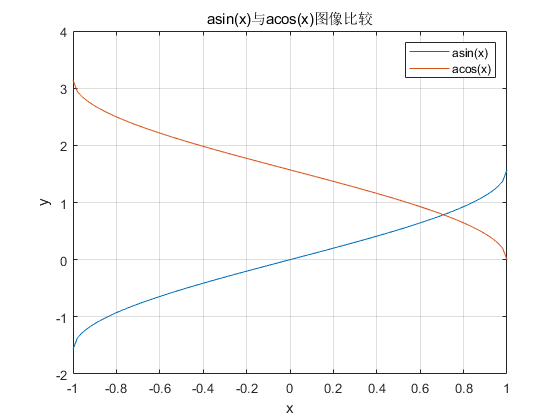图3

如图3所示，y轴的间隔即使由matlab自动控制的，再看图4，使用set(gca,'ytick',ylabel_pos,'yticklabel',ylabel_str);得到以下图像。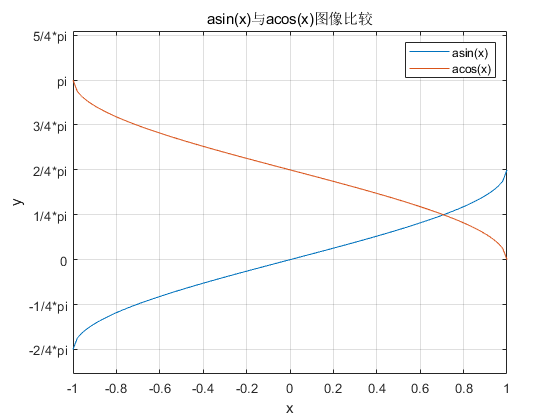图4

如图4所示，y轴的间隔和标注即设成了我们想要的。

2.关于set(gca,'ytick',ylabel_pos,'yticklabel',ylabel_str);的说明，如下。

ylabel_pos = -pi:pi/4:2*pi; %按照自己想要的间隔分
ylabel_str =
{'-pi','-3/4*pi','-2/4*pi','-1/4*pi','0','1/4*pi','2/4*pi','3/4*pi','pi','5/4*pi'};%每个间隔点的自定义字符表示
set(gca,'ytick',ylabel_pos,'yticklabel',ylabel_str);

set语句必须放在画图函数的后面,不然就无法使y轴的间隔和标注即设成了我们想要的，而还是会由matlab自动确定。

也可以 set(gca,'ytick',ylabel_pos);  set(gca,'yticklabel',ylabel_str);

以上两句与set(gca,'ytick',ylabel_pos,'yticklabel',ylabel_str);等价。

展开全文• ## MatLab绘图命令

千次阅读 2015-10-13 09:44:16
a=linspace(1,2,10) plot(a,'--pr','linewidth',1.5,'MarkerEdgeColor','r','MarkerFaceColor','m','MarkerSize',10) legend('a','Location','best')...title('a','FontName','Times New Roman','FontWeight','Bold','Fplot
• 常用Matlab作图命令 1.概率统计作图 1.1绘出正态分布的密度函数曲线 x=-5:0.1:5; y=normpdf(x,0,1; z=normpdf(x,0,2; plot(x,y,x,z) gtext'N(0,1) gtext'N(0,2) title'正态分布密度曲线) 1.2绘出t-分布的密度函数...
• 本文用来记录在matlab绘图过程中，常用的指令，仅供大家参考，不定时更新。 指定标题的位置及大小： title(‘txt’,‘position’,[-120,0],‘FontSize’,16); 坐标轴用Latex解释器： xlabel({time /μ\muμs’},...
• ## Matlab 基本画图命令

千次阅读 2018-09-03 08:18:32
Matlab中画图是对坐标、线条等如何控制 解决方案： 代码如下 x=0:pi/100:2*pi; y1=sin(x); y2=sin(x-0.25); plot(x,y1,'b-',x,y2,'r--') //定义图的线条样式和颜色 title('练习图') //图名 xlabel('x轴...
• [FileName,PathName,FilterIndex] =uigetfile(FilterSpec,DialogTitle,DefaultName)FileName：返回的文件名PathName：返回的文件的路径名FilterIndex：选择的文件类型FilterSpec：文件类型设置DialogTitle：打开...
• 今天，简单分享几个Matlab二维画图常用的命令，让你也可以画出漂亮的图形。单坐标轴曲线绘制绘图一般分为三步：数据准备、画图命令、增加修饰。1.数据准备一般可以直接在Matlab工作空间建立数据，或者通过命令导入...
• 1. 问题描述MATLAB基本的子图绘制功能，...2. 技术背景采用MATLAB的subplot命令。3. 解决方案MATLAB中子图的排列顺序如下，要分块绘制子图，只需指定预期占用的子图空间。如问题描述中的图像，可以采用subplot(4,...
• 使用Matlab进行数据分析，经常需要用到plot命令将数据可视化。Python提供一个非常好用的库：Matplotlib(Python 2D绘图库)，它提供了类似matlab的画图接口，包括：figure：图像的窗口，即画图区域；plot：画图命令；...
• ﻿uigetfile命令的应用此函数的用法为[FileName,PathName,FilterIndex] = uigetfile(FilterSpec,DialogTitle,DefaultName)FileName：返回的文件名PathName：返回的文件的路径名FilterIndex：选择的文件类型...
• uigetfile命令的应用此函数的用法为[FileName,PathName,FilterIndex] =uigetfile(FilterSpec,DialogTitle,DefaultName)FileName：返回的文件名PathName：返回的文件的路径名FilterIndex：选择的文件类型FilterSpec：...
• 今天，简单分享几个Matlab二维画图常用的命令，让你也可以画出漂亮的图形。单坐标轴曲线绘制绘图一般分为三步：数据准备、画图命令、增加修饰。1.数据准备一般可以直接在Matlab工作空间建立数据，或者通过命令导入...
• matlab中uigetfile命令的应用 uigetfile命令的应用 此函数的用法为 [FileName,PathName,FilterIndex] = uigetfile(FilterSpec,DialogTitle,DefaultName) FileName：返回的文件名 PathName：返回的文件的路径名 ...
• [FileName,PathName,FilterIndex] =uigetfile(FilterSpec,DialogTitle,DefaultName)FileName：返回的文件名PathName：返回的文件的路径名FilterIndex：选择的文件类型FilterSpec：文件类型设置DialogTitle：打开...
• 本示例说明如何使用hold功能在同一坐标系下组合绘图，以及如何使用tiledlayout功能在图形中...但是，您可以使用该hold on命令在同一轴上合并多个图。例如，绘制两条线和一个散点图。然后将保持状态重置为关闭。x ...
• MATLAB的subplot函数，让我们可以在一个图中画多张图，大多数情况下，系统自动平均分配的大小非常合适。但有时候，我们需要几个子图有不同的大小，或者是需要不均匀的位置，例如：函数图的三个视角立体频谱图和声波...
• selpath = uigetdir(path,title) uigetfile file = uigetfile [file,path] = uigetfile [file,path,indx] = uigetfile ___ = uigetfile(filter) ___ = uigetfile(filter,title) ___ = uigetfile(filter,titl
• matlab学习文档 数字图像处理 1、读入一幅RGB图像，变换为灰度图像和二值图像，并在同一个窗口内分成三个子窗口来分别显示RGB图像和灰度图像，注上文字标题。 a = imread('D:\study\third_down\ImageProcessing\...计算机视觉 边缘检测
• 经过大佬的指导，我用MATLAB画的图是这样的 在上一篇《如何利用MATLAB画出不被导师嫌弃的图像》中，介绍了一些MATLAB的基本绘图命令，有些MATLAB的大佬对我的文章提供了宝贵的意见，在这里首先感谢几位大佬的宝贵...
• Matlab常用画图调整 %单y轴 plot(t*1e+9,abs(iGG)/max(abs(iGG'k'linewidth,2; axis([-5,5,0,1]) xlabel'时间/ns; ylabel'幅度/a.u; set(get(gca'title'FontSize,10'FontName'宋体%设置标题字体大小字型 set(get(gca...
• 1、进入数据目录cd('F:\workspace\querytime')2、加载数据文件 a=load('result2018-03-14 15-05.csv')3、矩阵数据处理,下标从1开始a(:,1)表示第...title('平均请求时间与每秒发包数的关系') xlabel('平均请求时间')...
• 1、平面画图（plot） >>x = [0:0.01:10]; >>y = cos(x); >>plot(x, y), grid on %画网格 >>xlabel('x'),ylabel('cos(x)'), title(‘三角函数’); %横坐标，纵坐
• Matlab可以利用简单的命令来改变坐标轴标签的角度、字体、大小、内容等，请不要被那些过时的帖子浪费时间了！ 比如下面的这几个帖子： matlab中figure的坐标轴label、title、xticklabel的旋转 MATLAB 画柱状图（/...
• 第一章 MATLAB 简介 及基础知识;第二章 MATLAB基础知识 ;三环境;四语言命令;例MATLAB图标的绘制;... axis off,axis([1 n 1 n -.2 .8],view(-37.5,30) title'MATLAB 6.X的图标;五MATLAB的联机帮助;六桌面环境及窗口
• 示例： a=linspace(1,2,10) plot(a,'--pr','linewidth',1.5,'MarkerEdgeColor','r','MarkerFaceColor','m','MarkerSize',10) legend('a','Location'...title('a','FontName','Times New Roman','FontWeight','Bol...# title命令matlabmatlab 订阅